# Porting TensorFlow models to the IPU¶

## Introduction¶

This document is a practical guide to porting TensorFlow™ models to the Poplar SDK for running on the IPU. It is assumed that the reader is aware of the document Targeting the IPU from TensorFlow, which serves as the primary introduction to developing TensorFlow models for the IPU. It provides not only a conceptual introduction to developing models from the framework level, but also details a number of specific facets of the TensorFlow-to-Poplar API that are pivotal to running models on the IPU. In what follows, this general guide will be referred to as various topics arise.

This document will in turn focus on some of the practical considerations of developing a model for the IPU and provide some guidance on best practices. In doing so, it will attempt to identify those key elements that assist the developer in transitioning to using TensorFlow on the IPU.

Note

This document currently applies to the Graphcore port of TensorFlow 1.15.

The scope of this document includes:

• How to approach porting in general and which questions to ask upfront

• Code examples that highlight IPU-specific API functions

• Preliminaries in terms of bash environment setup and Python import statements

• The role of infeeds and outfeeds in boosting computational throughput

• Profile report generation by which the developer can identify compute or memory inefficiencies

• Sharding, a model parallel method of segregating a graph across multiple IPUs

• The IPUEstimator, a TensorFlow abstraction that facilitates session handling

A working knowledge of the above elements will allow the framework developer to take their first steps in transitioning to the IPU/Poplar/TensorFlow compute stack.

Note

Graphcore terminology

We use the term sharding to mean splitting a graph across multiple IPUs so they execute at the same time (basic model parallelism).

We use the term pipelining to mean splitting a graph into multiple computational stages, where stages are executed in parallel across multiple IPUs (another form of model parallelism).

We use the term replication to mean duplicating a graph over multiple IPUs (data parallelism).

An example application that demonstrates the use of sharding, pipelining and replication in the context of training CNNs including ResNet and ResNeXt is available in the Graphcore examples repository on GitHub: https://github.com/graphcore/examples/tree/master/applications/tensorflow/cnns/training

## How to transfer an existing code base¶

### Preliminary analysis¶

Before porting TensorFlow models to the IPU, it can be helpful to first assess the problem at hand.

What is the model size? One IPU has a memory size of 304 MiB. Will I use the IPU for training or inference? Training can require more memory. Here, it is also good to get an idea about the minimal batch size, for example for batch normalization. Depending on these estimates, it is possible to get an idea of whether the whole model will easily fit onto one IPU or if a setup with multiple IPUs is necessary.

An analysis of existing code can indicate whether more than one IPU is necessary too. Some programs distribute different tasks onto different devices like having training and inference in parallel for reinforcement learning applications. Some programs distribute the same task on multiple devices (data parallel) and others distribute one task across several devices (model parallel). Hence, a similar scheme might have to be used with multiple IPUs.

Additionally, it is important to assess the suitability of the existing code for the porting. On the one hand, if the code is mainly based on the estimator’s train, evaluate, and predict functions, replacing it by the `IPUEstimator` might be all that is required.

Similarly, when the `tfcompile` tool is used for code optimization together with feeds and fetches (instead of the estimator approach), replacing the compiler and data streams with the respective IPU counterparts could ease the transition.

If the code is very specialized for a device (for example TPU estimator or other TPU specific functions) and not general at all, it is maybe better to start with the existing model code and refactor the surrounding code. Graphcore provides a separate repository with plenty of code examples https://github.com/graphcore/examples. It is worth exploring these and to check if a similar problem is solved with a slightly different model.

The IPU documentation includes a list of supported operations (depending on the related data types) - see https://www.graphcore.ai/docs/targeting-the-ipu-from-tensorflow#document-supported_ops. If the code contains operations that are not yet supported, either a new Poplar implementation will be required, or the respective part has to be processed on the CPU which is achieved by scoping.

### Planning the transfer¶

The following guidelines are only an idea. There are multiple non-exclusive paths which can be taken.

If the model is sufficiently small and the code looks like a transfer is straightforward, it makes sense to start with the full model. If the model is very big or contains components that are not supported by the IPU, it makes sense to first start with a small version of the model.

A similar approach is useful when looking at the code base. If the implementation is rather simple, a direct change will probably work. Otherwise, it is good to break it down into smaller development examples. Since more complex code should come with meaningful unit tests, tests are probably a good starting point.

A different approach is to use our repository of examples. Instead of changing an existing code base, it might be easier to just transfer the model of interest to an existing example.

### Next steps¶

The first step after planning is to port the model and work through common initial issues like operations that have to be mapped onto the CPU (see Scoping and determining unsupported operations), or wrong input datatypes for the estimator because the original code provides iterators instead of datasets or feeds. If no estimator is used, it is recommended to use feeds to enable optimal communication between host and IPU (see ResNext inference example).

The second step is to profile the code to understand potential bottlenecks that might impact processing speed or memory consumption. One approach to explore bottlenecks outside of the IPU is the intrinsic Python profiler (`cprofile`). In Generating a report, we describe how the IPU can be efficiently profiled.

Thirdly, if a smaller model was used for getting started, it is now time to scale the model with potentially further profiling. There are two approaches for scaling, if the model easily fits on one IPU, the batch size can be increased and multiple IPUs can process the data in parallel. In other cases, it is better to distribute the IPU model. This concept of sharding is explained in Sharding: a model parallel approach.

## ResNext inference example¶

When being introduced to a new API, it is often helpful to have a working example of code to get a general overview of the key elements involved. A particular model which is useful to review given its simple topology is ResNeXt.

ResNext is an Inception inspired model based on ResNet with repeated computational blocks interspersed with residual connections. Its primary distinguishing characteristic is the use of group convolutions in its module compute structure. Group convolutions, as opposed to conventional convolutional layers, partition the output channels of the operation into segregated groups. The number of segregated groups is referred to as the model’s cardinality, which the authors state allows for more robust syntax extraction by allowing for more complex transformations. An illustration of cardinality is given in the figure below, where each of the [256, 1, 1, 4] streams in the graph represent a distinct convolution set, while the structure as a whole is a group convolution.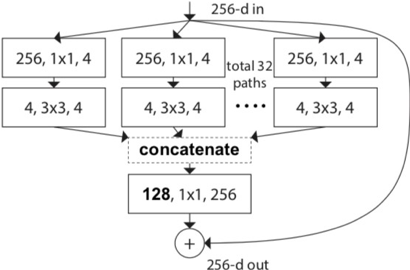ResNext cardinality expressed via group convolutions

### Abridged sample code¶

In the code sample that follows, only those facets that are specific to the IPU API are explicitly documented, while other general items of TensorFlow development are identified but mostly omitted. A full working version of the code can be found in ResNeXt full code example. Omissions are indicated by … and accompanied by comments to define the nature of what has been redacted.

 ``` 1 2 3 4 5 6 7 8 9 10 11 12 13 14 15 16 17 18 19 20 21 22 23 24 25 26 27 28 29 30 31 32 33 34 35 36 37 38 39 40 41 42 43 44 45 46 47 48 49 50 51 52 53 54 55 56 57 58 59 60 61 62 63 64 65 66 67 68 69 70 71 72 73 74 75 76 77 78 79 80 81 82 83 84 85 86 87 88 89 90 91 92 93 94 95 96 97 98 99 100 101 102 103 104 105 106 107 108 109 110 111 112 113 114 115 116 117 118 119 120 121 122 123 124 125 126 127 128``` ``` import tensorflow as tf ... # Various additional import statements # IPU specific imports from tensorflow.python import ipu from tensorflow.compiler.plugin.poplar.ops import gen_ipu_ops from gcprofile import save_tf_report # Create a dataset for in-feed interface into session call def create_input_data(batch_size=1, height=224, width=224, channels=4): # Synthetic input data follows NHWC format input_data = np.random.random((batch_size, height, width, channels)) input_data = tf.cast(input_data, DTYPE) ds = tf.data.Dataset \ .range(1) \ .map(lambda k: {"features": input_data}) \ .repeat() \ .prefetch(BATCHES_PER_STEP).cache() return ds ... # Various layer wrappers required by model definition, # (e.g. convolution, max pool) # Group convolution definition def group_conv(x, ksize, stride, filters_in, filters_out, index=0, groups=1, dtype=tf.float16, name='conv'): with tf.variable_scope(name, use_resource=True): # Define a weight variable W = tf.get_variable("conv2d/kernel" + str(index), shape=[ksize, ksize, filters_in.value / groups, filters_out], dtype=dtype, trainable=True, initializer=tf.variance_scaling_initializer()) # Implicit group confolution since channels of W are fraction of x return tf.nn.conv2d(x, filters=W, strides=[1, stride, stride, 1], padding='SAME') def group_conv_block(x, first_stride, filters, count, name='', cardinality=4): ... # Define the modular group convolution block as described in the paper return x # Define the ResNext model def resnext101_model(): def body(features): with tf.variable_scope("VanillaResNeXt"): ... # Elements of model definition output = fc(x, num_units_out=1000) outfeed = outfeed_queue.enqueue(output) return outfeed return tf.python.ipu.loops.repeat(n=BATCHES_PER_STEP, body=body, infeed_queue=infeed_queue) if __name__ == '__main__': # Report flags REPORT = False # no simulation IPU_MODEL = False ... # Various additional variables # Create input data using randomized numpy arrays dataset = create_input_data(batch_size=BATCH_SIZE, height=224, width=224, channels=4) if IPU_MODEL: os.environ['TF_POPLAR_FLAGS'] = "--use_ipu_model" # Setup infeed queue with tf.device('cpu'): infeed_queue = ipu.ipu_infeed_queue.IPUInfeedQueue(dataset, feed_name="inference_infeed") # Setup outfeed outfeed_queue = ipu.ipu_outfeed_queue.IPUOutfeedQueue(feed_name="outfeed") # Compiles graph and targets IPU(s) with ipu.scopes.ipu_scope('/device:IPU:0'): res = ipu.ipu_compiler.compile(resnext101_model, inputs=[]) # Capture IPU event trace for reporting if REPORT: with tf.device('cpu'): report = gen_ipu_ops.ipu_event_trace() # Setup IPU configuration and build session cfg = ipu.utils.create_ipu_config(profiling=REPORT, use_poplar_text_report=False, profile_execution=REPORT, max_report_size=1727937556, always_rearrange_copies_on_the_host=True, disable_graph_convolution_caching=False) cfg = ipu.utils.set_convolution_options(cfg, convolution_options={"availableMemoryProportion": "0.3"}) cfg = ipu.utils.set_ipu_model_options(cfg, compile_ipu_code=False) cfg = ipu.utils.auto_select_ipus(cfg, num_ipus=1) ipu.utils.configure_ipu_system(cfg) ipu.utils.move_variable_initialization_to_cpu() outfeed = outfeed_queue.dequeue() # Create a session initiation and run the model with tf.Session() as sess: fps = [] latency = [] sess.run(infeed_queue.initializer) sess.run(tf.global_variables_initializer()) # Warm up print("Compiling and Warmup...") start = time.time() sess.run(res) outfeed = sess.run(outfeed) if REPORT: rep_out = sess.run(report) # Create a gc-profile report if GC_PROFILE: save_tf_report(rep_out) else: # Create a written report rep = ipu.utils.extract_all_strings_from_event_trace(rep_out) with open("test_report.txt", "w") as f: f.write(rep) sess.close() else: for iter_count in range(NUM_ITERATIONS): print("Running iteration for benchmarking: ", iter_count) sess.run(res) sess.run(outfeed) ... # Various summary statistics ```

In the following three sections, we review specific elements of the code presented, using the line numbers to identify the pertinent code elements.

### Preliminaries: getting up and running¶

Before running the script, it is necessary to ensure a Poplar SDK has been downloaded and extracted on an IPU-enabled platform and that the environment variables are set appropriately. For bash, the environment variables are set in `.bashrc`, and a sample configuration would be:

 ```1 2 3 4 5 6``` ```  source /mnt/data/username/gc_drivers-ubuntu_18_04_specific_sdk/enable.sh source /mnt/data/username/poplar-ubuntu_18_04_specific_sdk/enable.sh # Export statements export TMPDIR=/mnt/data/username/tmp/ export TF_POPLAR_FLAGS="--executable_cache_path=/mnt/data/username/ipu_cache/" export POPLAR_LOG_LEVEL=INFO ```

The first two entries load the IPU-specific drivers and Poplar SDK backend which TensorFlow will reference through its API. After downloading a new SDK a Graphcore wheel file is included, in the release folder, that needs to be installed in the specific Python environment used for development. Once the above two `enable.sh` scripts are sourced, all that is required is to use pip to install TensorFlow, for example:

``` pip3 install gc_tf_wheel_file_for_specific_sdk-linux_x86_64.whl
```

This will allow Graphcore’s TensorFlow release to be used from the Python environment. More information can be found in the getting started guide, which can be found on the support site https://www.graphcore.ai/support.

Moving on to the export statements, Poplar in combination with TensorFlow stores computed graphs and their compilation. It is important to make sure there is enough dedicated space for it, which is why the first export statement points to a temp scratch directory. The second export is a flag variable configuration command that is used for development. When the compiler optimizes the tile layout for convolutions, there can be a significant compile time. The caching makes sure that when you run the program again, that there is no recompiling. In addition, for repeated calls of `session.run` or `estimator.train`, it will speed up processing time after the first run. The last export item is to ensure a number of relevant items are logged from the Poplar compilation.

Returning to the sample code, lines 5 to 7 import the relevant libraries from the TensorFlow IPU API:

• The `ipu` library is the primary API library that provides a number of functional aspects including graph compilation, configuration of the graph compilation via the utils sub-library, and access to infeeds and outfeeds

• `gen_ipu_ops` allows for a trace event to be attached to a TensorFlow session run for profiling

• The `gc-profile` utility is imported so that the PopVision™ Graph Analyser tool can render a graphical interpretation of the run profile, a subject that is discussed in greater detail in Generating a report.

### Configuring the IPU¶

There are several configuration parameters that are made available to the Tensorflow application developer, and the document Targeting the IPU from TensorFlow provides valuable insight into these settings. Here, we review some that are frequently required and explain their role. From lines 87 to 96, the script sets the working configuration for the IPU. The parameters to `create_ipu_config` are:

``` profiling = REPORT,
use_poplar_text_report = False,
profile_execution = REPORT,
max_report_size = 1727937556,
always_rearrange_copies_on_the_host = True,
disable_graph_convolution_caching = False
```

These define if profiling is required, and whether the report is to be text or parsed for the PopVision Graph Analyser tool. The maximum size of the log file is then defined, and the role of host in arranging tensor copies, and if convolution caching (for speedup) is enabled. Note that the `always_rearrange_copies_on_the_host` parameter is experimental in Poplar SDK 1.1.

Line 93 sets the `availableMemoryProportion` for convolutions. This parameter represents the proportion of tile memory to be made available as temporary memory for convolutions - it can vary between 0 and 1.0. Less temporary memory allocated will result in a higher number of cycles to compute a given convolution task, but too much memory allocation may oversubscribe the tile. Profiling, discussed in the next section, will provide insight into how this parameter affects model compilation. Line 95 defines if the code needs to be compiled as an IPU emulator on the host, which is not required when running on hardware. Finally, line 96 determines how many IPUs are required to compile and run the model.

### Generating a report¶

In developing TensorFlow models for the IPU, it is critical to profile the compiled graph when it is deployed to hardware. A variety of key elements can be documented by doing so that include the total size of the compiled model, the tile balance of consumed memory, and the cycle counts of the various compute processes. There are two main techniques to view this profile: the first is via a text report, which consists of a log file that can be generated directly from the TensorFlow IPU API; the second is via the PopVision Graph Analyser tool which renders similar elements of a text report, but in a streamlined GUI that presents the pertinent information in a more visual way. The sample script includes the code elements required to create both types of profiles.

Generating the text report requires three distinct elements. First, the import of `gen_ipu_ops` on line 6 allows laying a trace event into the TensorFlow session. The trace itself is subsequently defined on lines 83 and 84, where from the CPU device, the variable report is instantiated as the IPU event trace. When an actual TensorFlow session is created, the report variable is submitted as a run target after the defined graph is run, which in the sample code is done on line 113. This will return an extracted event trace, which is then post-processed for readability on line 119 and written to file on line 121. The resulting `test_report.txt` file can then be opened in any text editor. Further details are available from the Targeting the IPU from TensorFlow guide. A sample of a generated text report is given here:

``` ...
Graph:
Number of vertices:        3,927,766
Number of edges:          11,613,021
Number of variables:       6,356,577
Number of compute sets:        2,458

Memory Usage:
Total for all IPUs:
Including Gaps:        204,636,388 B
Excluding Gaps:
By Memory Region:
Non-interleaved:   154,228,933 B
Interleaved:        45,100,049 B
Overflowed:                  0 B
Total:               199,328,982 B
By Data Type:
Not Overlapped
Variables:                           18,251,593 B
Program and Sync IDs:                       352 B
Internal Exchange Message Buffers:       51,088 B
Data Rearrangement Buffers:                 438 B
Constants:                            3,958,638 B
Host Exchange Packet Headers:           652,472 B
...
```

It is possible to create a graphical rendition of the data generated in a text report, making the information more accessible.

The `gc-profile` command line tool is used to generate data in a format that can be used by the PopVision Graph Analyser. Both these tools are available from the Graphcore download portal: https://downloads.graphcore.ai/.

To generate the parsed elements of the profile used by `gc-profile`, `save_tf_report` must be imported, which requires the installation of `gc-profile` in the Python environment. From there, the same report trace event variable generated for the text report, (on line 83 of the script), is then submitted to a `session.run` directly after running the compiled graph. The resulting extracted trace, `rep_out`, is then parsed directly by `save_tf_report`. From there, running profiling on a script can be done with:

``` gc-profile -i -v -d /target/directory/profile/logs -- python code.py
```

The resulting profiling data can be visualized with the PopVision Graph Analyser tool. There is information on the memory breakdown, the tile usage, graph structure, compute operations, and respective length of processing cycles among other elements of the trace. Two sample graphical renditions from the PopVision Graph Analyser tool are given below.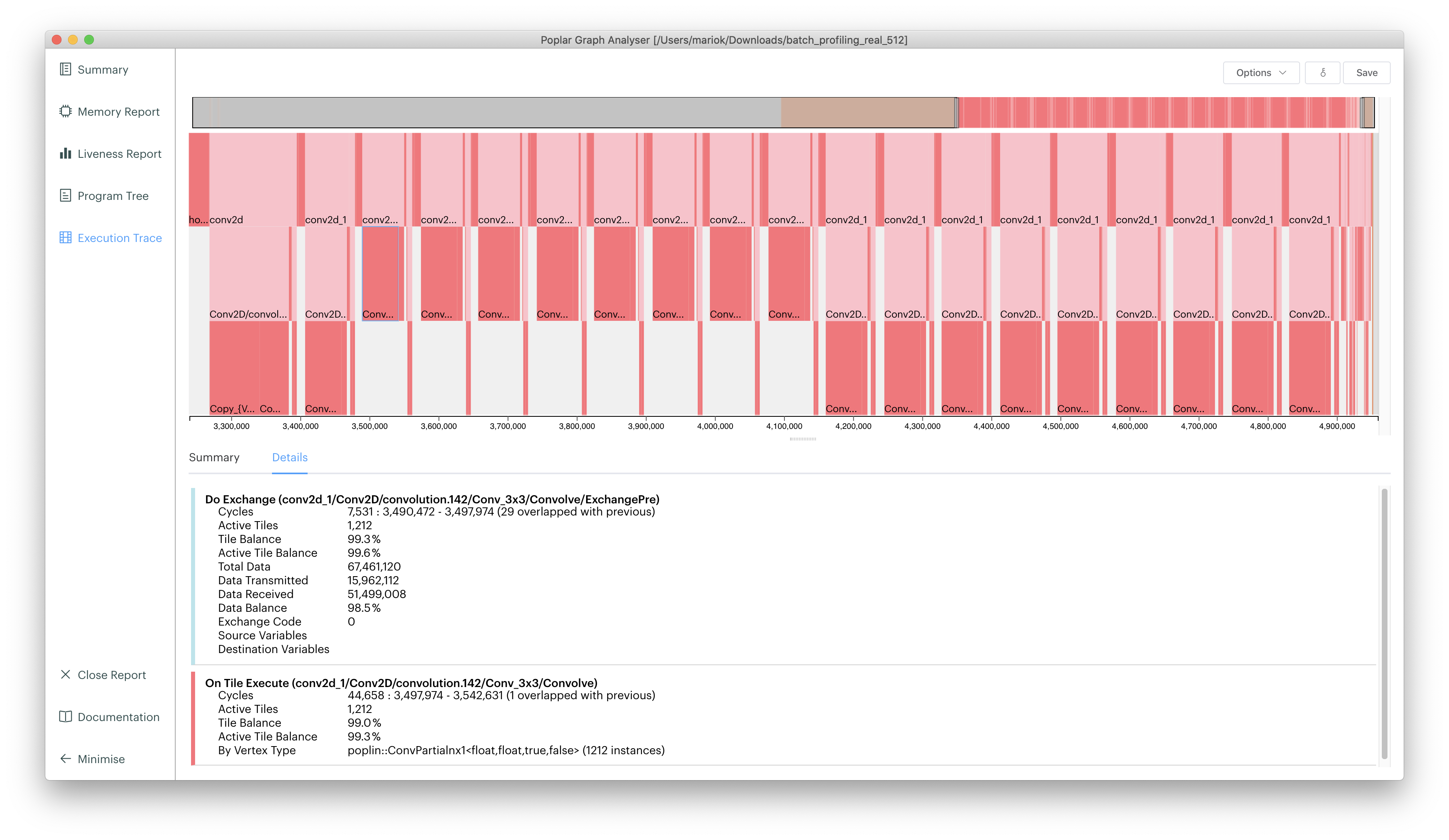Cycle breakdown of inference pipeline. The beginning section is waiting time for the pre-processing. The orange section is the data transfer. Both can be decreased using infeeds and outfeeds as described in Infeeds and outfeeds.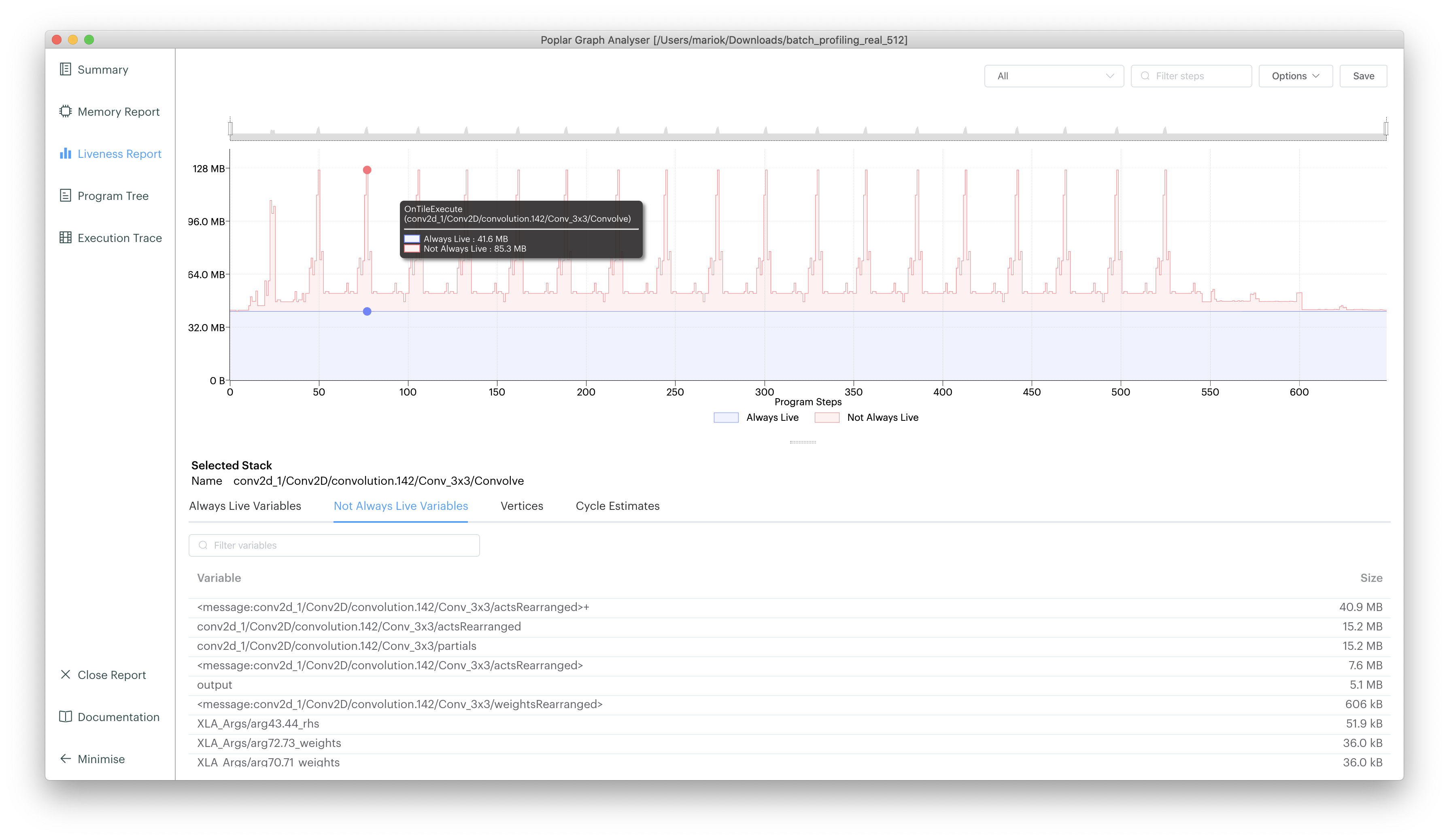Total memory usage of the IPU over processing time. The constant offset memory comes from the control code.

### Infeeds and outfeeds¶

Infeeds and outfeeds are framework constructions that allow data to be streamed directly into a TensorFlow session. This creates a significant boost in data throughput since the host-to-device transfer is an active stack making data available as required. The concept is illustrated below.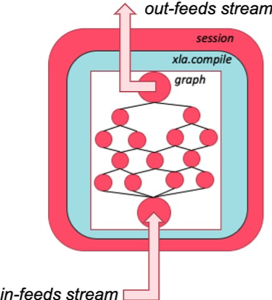The infeeds/outfeeds construction in relation to a session

The Targeting the IPU from TensorFlow guide provides a description of data feeds. Here, we mainly summarize the data feeds construction in the sample script.

The first step taken is to create a dataset as done on lines 10 to 20 where the input data (in this case a synthetic tensor of [batch size, h, w, channels] dimension), is packaged into the dataset `ds`. Lines 69 to 75 instantiate the infeed and outfeed queues.

Within the network definition, three additions are required. First, as done on line 46, a body(features) encapsulation is defined to hold the model definition. Secondly, the output of the model is fed into the outfeed construct on line 50. Finally, the return statement of the graph definition is a call to `ipu.loops.repeat` on line 52. On line 99, an outfeed dequeue is instantiated, which is the final preamble before a session definition. Within the session scope, an infeed queue is initialized on line 105, and after the `session.run` call to the compiled graph, the outfeed is dequeued. Data transfer thus follows a sequence of data upload into the infeed queue, session run of graph, data return via the outfeed queue.

Further aspects of this are presented within the guide and should be reviewed for greater insight.

## Sharding: a model parallel approach¶

A commonly used technique for porting models to the IPU is sharding, which is a concept derived from the model parallel paradigm where the same graph is segregated into separate components, or shards, and deployed to separate compute threads. In the current context, the graph is deployed to separate IPUs. Models that oversubscribe the memory of a single IPU can be deployed to multiple IPUs by breaking the network into smaller components and then communicating output activations of one shard to the input tensors of another shard.

The diagram below is taken from the Targeting the IPU from TensorFlow guide: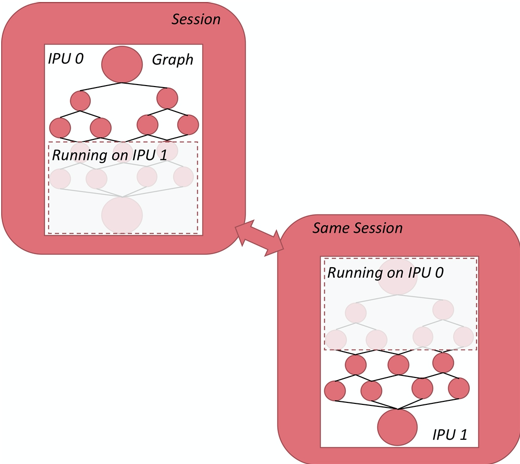The sharding concept

There are a number of ways to conduct sharding, and the Targeting the IPU from TensorFlow guide should be the first point of reference. Given here is a very abridged code example based on the script `simple_sharding.py` from Graphcore’s `examples` repository on GitHub. It presents a manual and automatic sharding code example:

``` from tensorflow.python.ipu import autoshard
...

# With sharding all placeholders MUST be explicitly placed on the CPU device:
with tf.device("cpu"):
pa = tf.placeholder(np.float32, , name="a")
pb = tf.placeholder(np.float32, , name="b")
pc = tf.placeholder(np.float32, , name="c")

# Put part of the computation on shard 1 and part on shard 2 for manual sharding
def manual_sharding(pa, pb, pc):
with scopes.ipu_shard(0):
o1 = pa + pb
with scopes.ipu_shard(1):
o2 = pa + pc
out = o1 + o2
return out

# Use autosharding to determine the points to best split up a network
def auto_sharding(pa, pb, p c):
with autoshard.ipu_autoshard():
o1 = pa + pb
o2 = pa + pc
out = o1 + o2
return out

def my_graph(pa, pb, pc):
with tf.device("/device:IPU:0"):
if opts.autoshard:
result = auto_sharding(pa, pb, pc)
else:
result = manual_sharding(pa, pb, pc)
return result

...
cfg = utils.auto_select_ipus(cfg, NUMBER_OF_SHARDS)
```

As can be seen from the code sample, in the manual sharding example, the graph is segregated by using the `scopes.ipu_shard` calls at the splice points in the graph. In the automatic sharding example, the graph as a whole is fed to the `ipu_autoshard` function and the API handles the splicing of the graph. Please refer to the `simple_sharding.py` script for further details of implementation.

### Limitations of sharding¶

Sharding technology simply partitions the model and distributes it on multiple IPUs. Multiple shards execute in series and cannot fully utilise the computing resources of the IPUs. As shown in the figure below, the model is partitioned into four parts and executed on four IPUs in series. Only one IPU is working at any one time.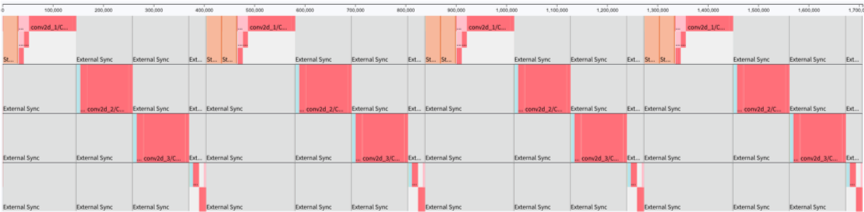Sharding profile

Sharding is a valuable method for use cases that need more control with replication, for example random forests, federated averaging and co-distillation. It can also be useful when developing/debugging a model, however for best performance pipelining should be used in most cases.

## Training with the estimator API¶

The TensorFlow estimator is a high-level API that encapsulates several common functions that are useful during the development of deep learning models. These functions include (but are not limited to) training and evaluation methods. They provide the primary interface between the user and the underlying model. Given the level of abstraction, it is somewhat of a departure from the session scope paradigm so common to many TensorFlow scripts and is reviewed as a separate topic. The Estimator is supported on Graphcore’s TensorFlow release.

There are two primary facets of the estimator: the `train` method and the `evaluate` method. In the `train` method, the user provides an input pipeline which is a function that is called for fetching a mini-batch from the training dataset. The number of iterations in the training loop can be specified by providing a steps parameter. The state of the model is captured in the checkpoint (.ckpt) file, which is stored in a specified model directory. The `evaluate` method is typically used for model quality assessment, where certain metrics are produced to quantify the performance of a model based on validation data. The model under evaluation is fetched via a specified checkpoint (.ckpt) file. Similar to the `train` method, the user is expected to provide the input pipeline function and the number of steps when the `evaluate` method is invoked.

### Instantiate an estimator for the IPU¶

The estimator is named `IPUEstimator` in the API. The following provides the essential arguments for instantiating an `IPUEstimator`:

• `config`: set the configuration of the estimator, which includes:

• IPU profiling configuration

• IPU selection configuration (number of IPUs to target, ID of IPU etc.)

• graph placement configuration: number of shards, number of replicas etc.

• logging configuration: parameters which control the logging frequency

• output configuration: directory of output checkpoint files

• `model_fn`: definition of the model function. Refer to https://github.com/tensorflow/docs/blob/master/site/en/r1/guide/custom_estimators.md#write-a-model-function for details on how to write this function.

• `model_dir`: directory to save model parameters, graph etc.

• `params`: hyperparameters to pass to the model function

• `warm_start_from`: optional path to checkpoint file to warm start from

### Abridged code sample for the estimator¶

The following is an abridged sample script that instantiates an estimator:

``` from tensorflow.python import ipu

def create_ipu_estimator(model_fn, model_dir, params):
# Create IPU configurations with profiling config spec
ipu_options = ipu.utils.create_ipu_config(
profiling=True,
use_poplar_text_report=False,
profile_execution=True)

#  IPU selection configuration
ipu_options = ipu.utils.auto_select_ipus(ipu_options,
num_ipus=params['num_devices'])

# Graph placement configuration
ipu_run_config = ipu.ipu_run_config.IPURunConfig(
iterations_per_loop=params['iterations_per_loop'],
ipu_options=ipu_options,
num_shards=1,
num_replicas=params['num_devices'],
autosharding=False)

# logging and output configuration
config = ipu.ipu_run_config.RunConfig(
ipu_run_config=ipu_run_config,
log_step_count_steps=params['log_interval'] ,
save_summary_steps=params['summary_interval'] ,
model_dir=model_dir)

# return an IPUEstimator instance
return ipu.ipu_estimator.IPUEstimator(
config=config,
model_fn=model_fn,
params=params)

# Instantiate an IPUEstimator
estimator = create_ipu_estimator(
model_fn,
model_dir=params['model_dir'],
params=params)
```

### Train and evaluate methods¶

Once an `IPUEstimator` is instantiated, the model can be trained and evaluated with the `train` and `evaluate` methods. These can be initiated as follows:

``` # partial is used to configure the training mode of the input function (input_fn)
train_input_fn = functools.partial(input_fn, params=params, is_training=True)

eval_input_fn = functools.partial(input_fn, params=params, is_training=False)

estimator.train(train_input_fn, steps=train_steps)
estimator.evaluate(eval_input_fn,
checkpoint_path=estimator.latest_checkpoint(),
steps=eval_steps)
```

For multiple epochs of training, you can calculate a number of steps according to the number of samples in the data set and the batch size. Then you invoke the `train` and `evaluate` methods in a loop of epochs as shown below:

``` for i in range(args.epochs):
print("Training epoch {}/{}".format(i, args.epochs))
estimator.train(train_input_fn, steps=train_steps)

estimator.evaluate(eval_input_fn,
checkpoint_path=n_est.latest_checkpoint(),
steps=eval_steps)
```

The `train_input_fn` and `evaluate_input_fn` parameters, which are supplied to the estimator methods, are the inputs to the pipeline. Their major functionality is to extract feature and label pairs from examples in the dataset. For more information about how these input pipelines can be composed, search for `input_fn` in the evaluate section of the following page:

https://www.tensorflow.org/api_docs/python/tf/estimator/Estimator#evaluate

### Profiling with an estimator on the IPU¶

Profiling the device side execution of an application can be done with `gc-profile`. In the following, we provide a simple code snippet for adding profiling capability to the estimator construct using a profiling hook:

``` from tensorflow.python.ipu.ipu_optimizer import CrossReplicaOptimizer
from tensorflow.python.ipu.ipu_estimator import IPUEstimator
...

class ProfilerHook(tf.train.SessionRunHook):
def begin(self):
from tensorflow.compiler.plugin.poplar.ops import gen_ipu_ops
self._report = gen_ipu_ops.ipu_event_trace()

def end(self, session):
raw_reports = session.run(self._report)
from gcprofile import save_tf_report
save_tf_report(raw_reports)

profiler_hook = ProfilerHook()

estimator.train(input_fn, hooks=[profiler_hook], max_steps=2)
```

## Scoping and determining unsupported operations¶

Porting a model to the IPU usually requires an explicit scoping call to define which part of the graph goes onto the IPU. In its simplest form, this means taking the model definition and scoping it in its entirety on the IPU:

``` with ipu_scope('/device:IPU:0'):
original_graph_definition
```

or as defined in lines 78-79 of the ResNext example in Abridged sample code:

 ```77 78 79``` ``` # Compiles graph and targets IPU(s) with ipu.scopes.ipu_scope('/device:IPU:0'): res = ipu.ipu_compiler.compile(resnext101_model, inputs=[]) ```

The `ipu_compiler.compile` wrapper is compiling the model graph and optimizes it for the IPU. The IPUEstimator, explained in Training with the estimator API, uses the same approach internally and does not require an explicit scoping or compile.

In most cases, most facets of the model definition can be scoped to run on the IPU, which leads to a simplified deployment process. If an underlying operation in the graph is unsupported, however, such an approach is not possible and TensorFlow will provide an error that elaborates on which operation cannot run on the IPU and which are the supported and unsupported data types. In certain cases, it is necessary to place a component of the graph on the host:

``` with tf.device('/device:CPU:0'):
not_supported_op
```

The `allow_soft_placement` option can also be used to place unsupported operations on the CPU. If this option is used when constructing the `Session` then there’s no need to explicitly place operations on the host:

``` with tf.Session(config=tf.ConfigProto(allow_soft_placement=True)) as sess:
```

## Checking availability and hardware reset¶

Using the `gc-monitor` command, you can always check the availability of IPUs on the host machine. If a process did not finish appropriately, it might still be active and block an IPU. You can use the process ID to get rid of this process. This is especially useful after a process crash, where the IPU might be left in a bad state and it is helpful to reset it, as shown below:

``` for i in 'seq 0 15'; do gc_reset -d \$i || continue; done
```

This code will not affect any reserved IPUs and running processes and is safe to use on any active IPU Servers.

## ResNeXt full code example¶

``` import tensorflow as tf
import os
import numpy as np
import time
import logging

# IPU Tensorflow specific imports
from tensorflow.python import ipu
from tensorflow.compiler.plugin.poplar.ops import gen_ipu_ops
from tensorflow_core.python.keras.backend import unique_object_name
from tensorflow.python.ipu.ops import normalization_ops
from typing import Any, Optional, Tuple, Union
# from gcprofile import save_tf_report
# import custom_layers as cl

def create_input_data(batch_size=1, height=224, width=224, channels=4):
"""
Create the input dataset for in-feeds

:param batch_size: size of the batches to process
:param height: height of input image
:param width: width of input image
:param channels:
:return: Constructed dataset
"""
# Synthetic input data follows NHWC format
input_data = np.random.random((batch_size, height, width, channels))
input_data = tf.cast(input_data, DTYPE)

# Prepare dataset for ipu_infeeds
ds = tf.data.Dataset \
.range(1) \
.map(lambda k: {"features": input_data}) \
.repeat() \
.prefetch(BATCHES_PER_STEP)
return ds

def conv(input_tensor: tf.Tensor,
kernel_size: Union[int, Tuple[int, int]],
filters_out: int,
stride: Optional[int] = 1,
dtype: Optional[Any] = tf.float16,
name: Optional[str] = None,
weight_suffix: Optional[str] = "kernel",
bias_suffix: Optional[str] = "conv/bias",
*_):
"""
Apply convolutional layer and optional bias on input tensor.

Args:
input_tensor: Input data
kernel_size: Filter size (assumes equal height and width)
filters_out: Number of output filters
stride: Stride of the filter
dtype: Data type of parameters
name: Optional name for this op
weight_suffix:
bias_suffix:

Returns: Output of convolution operator.
"""

# Assumes input in NHWC format.
filters_in = input_tensor.get_shape()[-1]
if isinstance(kernel_size, int):
w_shape = [kernel_size, kernel_size, filters_in, filters_out]
else:
w_shape = kernel_size + (filters_in, filters_out)
w_init = tf.contrib.layers.xavier_initializer(dtype=dtype)
if name is None:
name = unique_object_name("conv2d", zero_based=True)

name_scope = tf.get_default_graph().get_name_scope()
if name_scope not in ["", None]:
name = name_scope + "/" + name

with tf.get_default_graph().as_default():
with tf.variable_scope(name):
weights = tf.get_variable(weight_suffix,
shape=w_shape,
initializer=w_init,
dtype=dtype)

output_tensor = tf.nn.conv2d(input_tensor,
weights, [1, stride, stride, 1],
name=name)

b_shape = [filters_out]
b_init = tf.zeros_initializer()
with tf.variable_scope(name):
biases = tf.get_variable(bias_suffix,
shape=b_shape,
initializer=b_init,
dtype=dtype)
output_tensor += biases
return output_tensor

# Block definitions for ResNeXt
def input_block(x):
x = conv(x, kernel_size=7, stride=2, filters_out=64, name="conv1")
x = norm(x, training=False)
x = relu(x)
x = maxpool(x, size=3, stride=2)
return x

def maxpool(x, size=3, stride=2):
x = tf.nn.max_pool(x,
ksize=[1, size, size, 1],
strides=[1, stride, stride, 1],
return x

def reduce_mean(x, indices=(1, 2)):
x = tf.reduce_mean(x, reduction_indices=indices)
return x

def fc(x, num_units_out):
num_units_in = x.get_shape()
w_init = tf.contrib.layers.xavier_initializer(dtype=tf.float16)
b_init = tf.constant_initializer(0.0)

weights = tf.get_variable('weights',
shape=[num_units_in, num_units_out],
initializer=w_init,
dtype=tf.float16)
biases = tf.get_variable('biases',
shape=[num_units_out],
initializer=b_init,
dtype=tf.float16)

x = tf.nn.xw_plus_b(x, weights, biases)
return x

def norm(x, training=False):
x = tf.layers.batch_normalization(x,
fused=True,
center=True,
scale=True,
training=training,
trainable=training,
momentum=0.997,
epsilon=1e-5)
return x

def relu(x):
return tf.nn.relu(x)

def group_conv(x,
ksize,
stride,
filters_in,
filters_out,
index=0,
groups=1,
dtype=tf.float16,
name='conv'):
"""
Apply group convolutions by leveraging XLA implementation.

"""
with tf.variable_scope(name, use_resource=True):
W = tf.get_variable(
"conv2d/kernel" + str(index),
shape=[ksize, ksize, filters_in.value / groups, filters_out],
dtype=dtype,
trainable=True,
initializer=tf.variance_scaling_initializer())
return tf.nn.conv2d(x,
filters=W,
strides=[1, stride, stride, 1],

def group_conv_block(x, first_stride, filters, count, name='', cardinality=4):
"""
Group convolution block implementation.

:param x: Input tensor
:param first_stride: Initial tensor
:param filters: List of number of filters for various convolution blocks
:param count: Number of times block is repeated
:param name: Name of block
:param cardinality: Number of groups = outputchannels/cardinality
:return:
"""
for i in range(count):
shortcut = x
stride = (first_stride if (i == 0) else 1)

# First vanilla convolution
x = conv(x,
kernel_size=1,
stride=stride,
filters_out=filters,
name=name + str(i) + "_1",
dtype=tf.float16)
x = norm(x)
x = relu(x)

# Group convolution evaluation
x = group_conv(x,
ksize=3,
stride=1,
filters_in=x.get_shape()[-1],
filters_out=filters,
index=1,
name=name + str(i) + "_2",
groups=cardinality,
dtype=tf.float16)
x = norm(x)
x = relu(x)

# Second vanilla convolution
x = conv(x,
kernel_size=1,
stride=1,
filters_out=filters,
name=name + str(i) + "_3",
dtype=tf.float16)
x = norm(x)
if i == 0:
shortcut = conv(shortcut,
kernel_size=1,
stride=stride,
filters_out=filters,
name=name + str(i) + "skip",
dtype=tf.float16)
shortcut = norm(shortcut)
x = shortcut + x
x = relu(x)
return x

def resnext101_model():
"""
Define ResNext-101 network graph

:return:
"""
def body(features):
with tf.variable_scope("VanillaResNeXt"):
x = input_block(features)
x = group_conv_block(x,
first_stride=1,
filters=[128, 256],
count=3,
cardinality=CARDINALITY,
name='res2_')  # 112
x = group_conv_block(x,
first_stride=2,
filters=[256, 512],
count=4,
cardinality=CARDINALITY,
name='res3_')  # 224
x = group_conv_block(x,
first_stride=2,
filters=[512, 1024],
count=23,
cardinality=CARDINALITY,
name='res4_')  # 448
x = group_conv_block(x,
first_stride=2,
filters=[1024, 2048],
count=3,
cardinality=CARDINALITY,
name='res5_')  # 896
x = reduce_mean(x)
output = fc(x, num_units_out=1000)
outfeed = outfeed_queue.enqueue(output)
return outfeed

return tf.python.ipu.loops.repeat(n=BATCHES_PER_STEP,
body=body,
infeed_queue=infeed_queue)

if __name__ == '__main__':
print("ResNeXt-101 Inference")

# Report FLAG
REPORT = False
IPU_MODEL = False

# Number of steps
NUM_ITERATIONS = 5
BATCHES_PER_STEP = 1000

# Model
MODEL = 'ResNeXt-101'
CARDINALITY = 32
BATCH_SIZE = 4

# Precision
DTYPE = tf.float16

# Create input data using randomized numpy arrays
dataset = create_input_data(batch_size=BATCH_SIZE,
height=224,
width=224,
channels=4)

if IPU_MODEL:
os.environ['TF_POPLAR_FLAGS'] = "--use_ipu_model"

# Setup infeed queue
if BATCHES_PER_STEP > 1:
with tf.device('cpu'):
infeed_queue = ipu.ipu_infeed_queue.IPUInfeedQueue(
dataset, feed_name="inference_infeed")
else:
raise NotImplementedError("batches per step == 1 not implemented yet.")

# Setup outfeed
outfeed_queue = ipu.ipu_outfeed_queue.IPUOutfeedQueue(feed_name="outfeed")

# Compiles graph and targets IPU(s)
with ipu.scopes.ipu_scope('/device:IPU:0'):
res = ipu.ipu_compiler.compile(resnext101_model, inputs=[])

# Capture IPU event trace for reporting
if REPORT:
with tf.device('cpu'):
report = gen_ipu_ops.ipu_event_trace()

# Setup IPU configuration and build session
cfg = ipu.utils.create_ipu_config(profiling=REPORT,
use_poplar_text_report=False,
profile_execution=REPORT,
max_report_size=1727937556,
always_rearrange_copies_on_the_host=True,
disable_graph_convolution_caching=False)
cfg = ipu.utils.set_convolution_options(
cfg, convolution_options={"availableMemoryProportion": "0.3"})
cfg = ipu.utils.set_ipu_model_options(cfg, compile_ipu_code=False)
cfg = ipu.utils.auto_select_ipus(cfg, num_ipus=1)
ipu.utils.configure_ipu_system(cfg)
ipu.utils.move_variable_initialization_to_cpu()
outfeed = outfeed_queue.dequeue()

with tf.Session() as sess:
fps = []
latency = []
sess.run(infeed_queue.initializer)
sess.run(tf.global_variables_initializer())
# Warm up
print("Compiling and Warmup...")
start = time.time()
sess.run(res)
outfed = sess.run(outfeed)
duration = time.time() - start
print("Duration: {:.3f} seconds\n".format(duration))
if REPORT:
rep_out = sess.run(report)
#save_tf_report(rep_out)
rep = ipu.utils.extract_all_strings_from_event_trace(rep_out)
with open(
"reports/" + "resnext_c_" + str(CARDINALITY) +
"_report.txt", "w") as f:
f.write(rep)
sess.close()
else:
for iter_count in range(NUM_ITERATIONS):
print("Running iteration: ", iter_count)
# Run
start = time.time()
sess.run(res)
sess.run(outfeed)
stop = time.time()
fps.append((BATCHES_PER_STEP * BATCH_SIZE) / (stop - start))
logging.info(
"Iter {3}: {0} Throughput using real data = {1:.1f}"
" imgs/sec at batch size = {2}".format(
str(MODEL), fps[-1], BATCH_SIZE, iter_count))
latency.append(1000 * (stop - start) / BATCHES_PER_STEP)
logging.info(
"Iter {3}: {0} Latency using real data = {2:.2f} msecs "
"at batch_size = {1}".format(str(MODEL), BATCH_SIZE,
latency[-1], iter_count))

print("Average statistics over {0} iterations, excluding the 1st "
"iteration.".format(NUM_ITERATIONS))
print("-------------------------")
fps = fps[1:]
latency = latency[1:]
print(
"Throughput at bs={} of {}: min={}, max={}, mean={}, std={}.".
format(BATCH_SIZE, str(MODEL), min(fps), max(fps),
np.mean(fps), np.std(fps)))
print("Latency at bs={} of {}: min={}, max={}, mean={}, std={}.".
format(BATCH_SIZE, str(MODEL), min(latency), max(latency),
np.mean(latency), np.std(latency)))
```